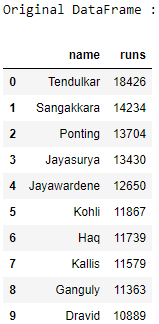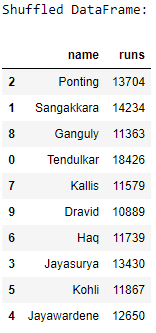Related Articles

# Pandas – How to shuffle a DataFrame rows

• Last Updated : 10 Jul, 2020

Let us see how to shuffle the rows of a DataFrame. We will be using the `sample()` method of the pandas module to to randomly shuffle DataFrame rows in Pandas.

Algorithm :

1. Import the `pandas `and `numpy `modules.
2. Create a DataFrame.
3. Shuffle the rows of the DataFrame using the `sample()` method with the parameter `frac` as 1, it determines what fraction of total instances need to be returned.
4. Print the original and the shuffled DataFrames.
 `# import the modules``import` `pandas as pd``import` `numpy as np`` ` `# create a DataFrame``ODI_runs ``=` `{``'name'``: [``'Tendulkar'``, ``'Sangakkara'``, ``'Ponting'``,``                      ``'Jayasurya'``, ``'Jayawardene'``, ``'Kohli'``,``                      ``'Haq'``, ``'Kallis'``, ``'Ganguly'``, ``'Dravid'``],``            ``'runs'``: [``18426``, ``14234``, ``13704``, ``13430``, ``12650``,``                     ``11867``, ``11739``, ``11579``, ``11363``, ``10889``]}``df ``=` `pd.DataFrame(ODI_runs)`` ` `# print the original DataFrame``print``(``"Original DataFrame :"``)``print``(df)`` ` `# shuffle the DataFrame rows``df ``=` `df.sample(frac ``=` `1``)`` ` `# print the shuffled DataFrame``print``(``"\nShuffled DataFrame:"``)``print``(df)`

Output :Attention geek! Strengthen your foundations with the Python Programming Foundation Course and learn the basics.

To begin with, your interview preparations Enhance your Data Structures concepts with the Python DS Course. And to begin with your Machine Learning Journey, join the Machine Learning – Basic Level Course

My Personal Notes arrow_drop_up